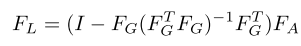# Dealing with matrix inversion efficiently

I have two matrix `X` and `Y`. Both are of dimension `[bs, hidden_size`].
I want to take inverse of matrix obtained by `torch.cat([X, Y], dim=1)`.

Pseudo inverse is not stable (docs say the same). I obtain inverse to deal with this. So I used a `Linear` layer of dimension `(hidden_size, batch_size//2)` to convert X and Y of dimensions `[bs, bs//2`]. After concat, I get a matrix of form `[bs, bs]`. The downside of this approach is that it requires me to use a `batch size = hidden_size/2` to perform well, otherwise the network doesn’t learn anything well.

Is there a mathematical elegant way you can suggest as a workaround ?

Hello kl!

Could you explain conceptually what meaning this matrix inverse
is supposed to have? Your construction seems odd.

As you have recognized, a matrix must be square to be invertible
(although not all square matrices are invertible, of course). But
your batch size (`bs`) is something of a 'technical" parameter that
doesn’t really have anything to do with your data or the structure of
to match `hidden_size / 2`. But how does your construction make
sense if it breaks when you change your batch size?

Best.

K. Frank

Thanks for taking interest. The matrix inversion is part of a formula that is supposed to project features orthogonally.`\$F_{G}\$` (comes from concatenation of two vectors) is not a square matrix, that’s why I make this modification.

The issue is, that my network performs much better if I reduce second dimensions of X and Y to half of what it was (768->384), in all other cases, it doesn’t learn anything. There might be better ways of projection, which I’ve not explored. (For instance, if bs=512, 768->512, then I don’t notice any improvement.)

This is an unstable method. The main issue is to perform inversion. That implies I need square matrices. That’s why I’m asking if there’s a better mathematical way to perform this.
Yes, I realize that my implementation is not batch size agnostic once model is trained. As a result, I am unable to train bigger models (because batch_size = hidden_size/2 won’t fit in memory).

So in general sense, what is a better way to perform inversion when the matrix is not square (`pinverse` isn’t stable) ?